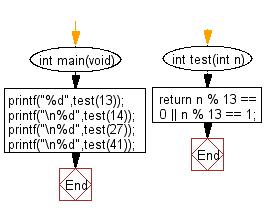﻿ C Program: A +ve number is a multiple of 13 or greater# C Exercises: Test whether a non-negative number is a multiple of 13 or it is one more than a multiple of 13

## C-programming basic algorithm: Exercise-19 with Solution

Write a C program to test whether a non-negative number is a multiple of 13 or it is one more than a multiple of 13.

C Code:

``````#include <stdio.h>
#include <stdlib.h>

int main(void){
printf("%d",test(13));
printf("\n%d",test(14));
printf("\n%d",test(27));
printf("\n%d",test(41));
}

int test(int n)
{
return n % 13 == 0 || n % 13 == 1;
}
``````

Sample Output:

```1
1
1
0
```

Flowchart:C Programming Code Editor:

What is the difficulty level of this exercise?

Test your Programming skills with w3resource's quiz.

﻿

## C Programming: Tips of the Day

Why doesn't a+++++b work?

printf("%d",a+++++b); is interpreted as (a++)++ + b according to the Maximal Munch Rule!.

++ (postfix) doesn't evaluate to an lvalue but it requires its operand to be an lvalue.

! 6.4/4 says the next preprocessing token is the longest sequence of characters that could constitute a preprocessing token"

Ref : https://bit.ly/3fdldUT